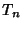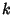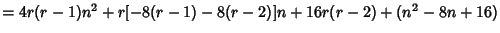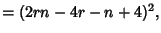## Polygonal NumberA type of Figurate Number which is a generalization of Triangular, Square, etc., numbers to an arbitrary-gonal number. The above diagrams graphically illustrate the process by which the polygonal numbers are built up. Starting with theth Triangular Number, then(1)

Now note that(2)

gives theth Square Number,(3)

gives theth Pentagonal Number, and so on. The general polygonal number can be written in the form(4)

whereis theth-gonal number. For example, takingin (4) gives a Triangular Number,gives a Square Number, etc.

Fermatproposed that every number is expressible as at most-gonal numbers (Fermat's Polygonal Number Theorem). Fermat claimed to have a proof of this result, although this proof has never been found. Jacobi, Lagrange(1772), and Eulerall proved the square case, and Gaußproved the triangular case in 1796. In 1813, Cauchyproved the proposition in its entirety.

An arbitrary numbercan be checked to see if it is a-gonal number as follows. Note the identity(5)
somust be a Perfect Square. Therefore, if it is not, the number cannot be-gonal. If it is a Perfect Square, then solving(6)

for the rankgives(7)

An-gonal number is equal to the sum of the-gonal number of the same Rank and the Triangular Number of the previous Rank.

See also Centered Polygonal Number, Decagonal Number, Fermat's Polygonal Number Theorem, Figurate Number, Heptagonal Number, Hexagonal Number, Nonagonal Number, Octagonal Number, Pentagonal Number, Pyramidal Number, Square Number, Triangular Number

References

Beiler, A. H. Ball Games.'' Ch. 18 in Recreations in the Theory of Numbers: The Queen of Mathematics Entertains. New York: Dover, pp. 184-199, 1966.

Dickson, L. E. History of the Theory of Numbers, Vol. 1: Divisibility and Primality. New York: Chelsea, pp. 3-33, 1952.

Guy, K. Every Number is Expressible as a Sum of How Many Polygonal Numbers?'' Amer. Math. Monthly 101, 169-172, 1994.

Pappas, T. Triangular, Square & Pentagonal Numbers.'' The Joy of Mathematics. San Carlos, CA: Wide World Publ./Tetra, p. 214, 1989.

Sloane, N. J. A. Sequence A000217/M2535 in An On-Line Version of the Encyclopedia of Integer Sequences.'' http://www.research.att.com/~njas/sequences/eisonline.html and extended entry in Sloane, N. J. A. and Plouffe, S. The Encyclopedia of Integer Sequences. San Diego: Academic Press, 1995.